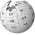# MapReduce您可以在Wikipedia上了解到此条目的英文信息 MapReduce Thanks, Wikipedia.

The Google MapReduce framework is implemented in C++ with interfaces in Python and Java.

## 简介

^ "我们的灵感来自lisp和其他函数式编程语言中的古老的映射和化简操作." -"MapReduce:大规模集群上的简单数据处理方式"

## 实现

In general, these functions could be defined as:

```List2 map(Functor1, List1);
Object reduce(Functor2, List2);
```

The general form of the map() function is:

```map(function, list) {
foreach element in list {
v = function(element)
}
} // map
```

The general form of the reduce() function is:

```reduce(function, list, init) {
result = init
foreach value in list {
result = function(result, value)
}
}
```

Java的方案中涉及 Xen , Terracotta , Mule 等技术

## Ruby

Skynet Ruby MapReduce Framework

## Erlang

• Apache CouchDB uses a MapReduce framework for defining views over distributed documents

## Python

Python 提供了map() 和 reduce() 函数, 是否能悟出些什么 :)

zip(list1, list2)”是把一对序列变成元素对序列的便捷方式

```>>> seq = range(8)
>>> def square(x): return x*x
...
>>> zip(seq, map(square, seq))
[(0, 0), (1, 1), (2, 4), (3, 9), (4, 16), (5, 25), (6, 36), (7, 49)]

```

reduce(func, sequence)" 返回一个单值，它是这样构造的：首先以序列的前两个元素调用函数，再以返回值和第三个参数调用，依次执行下去。

```>>> def add(x,y): return x+y
...
55
```